# Solving the Korteweg-de Vries equation

The Korteweg-de Vries equation (KdV for short) is a type of nonlinear partial differential equation (PDE) which is commonly used to model the behaviour of waves in shallow water. Despite it being nonlinear, it is a PDE that possesses a special set of analytic solutions known as “travelling wave solutions”. Here, we will look at one of those analytic solutions and how it is derived using coordinate transformations.

We shall begin with the standard KdV equation in 1 spatial dimension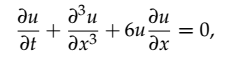Equation 1: KdV equation in 1D

where the solution u(x,t) represents the vertical displacement of water molecules at each position x and time t along a horizontal surface. To find a solution to this equation, we introduce a new coordinate

which acts as a “moving frame” (at a constant speed c, to be determined later). Then, using the chain rule (as explained in my previous story “Travelling wave solutions to partial differential equations”) we arrive at the transformed equation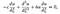Equation 2: KdV in the new coordinate system

Note how this new equation is an ODE (e.g. the derivatives only depend on one variable as opposed to two). We can also write this as

which allows us to integrate both sides

Now, multiplying both sides by the first derivative of u yields

and using the chain rule of differentiation again we get

and finally integrating both sides again yieldsEquation 3: transformed KdV equation in. terms of a single derivative

This is basically the same equation as before except we now only have one derivative term, everything else is some power of the solution u. At first glance this may not seem any easier to solve than our original KdV equation, but this has in fact a set of solutions in terms of “elliptic functions” for arbitrary constants A and B. We will however for the moment being focus on the special case A = B = 0 (the great thing about travelling wave solutions is we often can play around with the arbitrary constants to gain some insight), which yields the simplified ODE

Here, we can use separation of variables to obtain

and using a standard table of integrals for the left-hand side we obtain the solution in the original coordinate system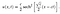Equation 4: special solution to the KdV equation

This solution is known as a “soliton” or solitary wave, since it propagates at a constant speed c whilst retaining its characteristic shape. Although this solution is very constrained in terms of the initial conditions we can use for the PDE, it is exact, and is very useful for testing numerical algorithms used for this equation.

A simple MATLAB code which plots this function at different times t is shown below:

% Input parameters

L = 20;

T = 4;

x = linspace(0,L,201);

t = linspace(0,T,301);

c = 0.1;

% Analytic solution

step = 30;

[X1,T1] = meshgrid(x,t(1:step:end));

u = @(x,t) c/2*sech( sqrt(c)/2*(x — c*t) ).²;

% Plots

az_angle = 30;

el_angle = 60;

FS = ‘\fontname{Palatino} ‘;

figure(1);

set(gcf,’color’,’w’);

pp = waterfall(X1,T1,u(X1,T1)); grid off;

pp.EdgeColor = ‘k’;

pp.LineWidth = 2;

view(az_angle,el_angle);

xlabel([FS ‘x (m)’]);

ylabel([FS ‘t (s)’]);

zlabel([FS ‘u(x,t)’]);

set(gca,’FontSize’,18);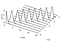Figure 1: Soliton solution to the KdV equation using c = 6

As we can see in Figure 1, the multiple frozen snapshots of u(x,t) at different times show that the soliton propagates to the right along the x-axis at a constant speed, as expected from the travelling wave solution. What is interesting about this soliton solution in Eq(4) is that both the amplitude of the wave and the width of the wave are controlled by the speed of propagation c. For example, if I bring down c = 6 to c = 0.1, I produce the plot in Figure 2. In this case, the wave is barely moving within this time frame, but we can see its width has significantly increased. So what does this tell us about the behaviour of shallow water waves? It basically suggests that as the wave moves faster, it can only retain its stable “constant shape” without breaking by becoming narrower and narrower, which is consistent with what we would observe in nature.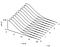Figure 2: Soliton solution to the KdV equation for c = 0.1

Oscar is a physicist, educator and STEM enthusiast. He is currently finishing a PhD in Theoretical Physics with a focus on photonics and stochastic dynamics.

## More from Oscar Nieves

Oscar is a physicist, educator and STEM enthusiast. He is currently finishing a PhD in Theoretical Physics with a focus on photonics and stochastic dynamics.